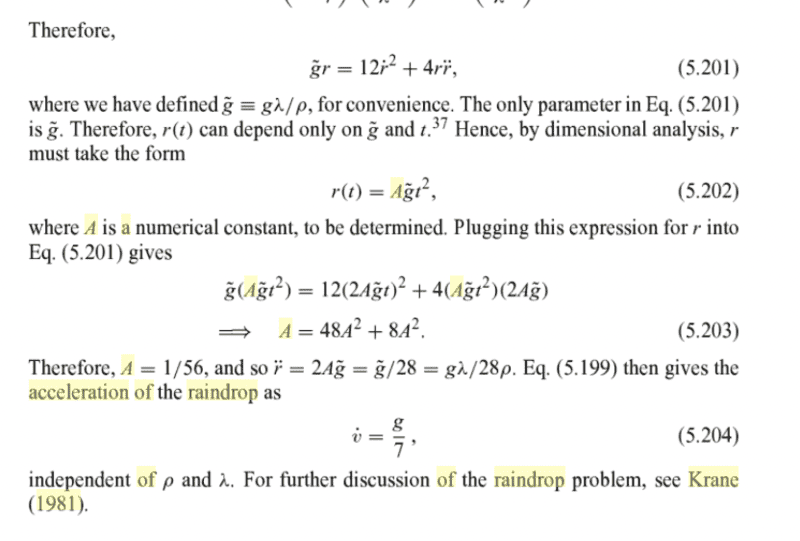# Solving a 2nd order non-linear DE by dimensional analysis/observation

• phantomvommand
phantomvommand
Homework Statement
See picture below
Relevant Equations
Dimensional analysis
Solving DEThe top most 2nd order non-linear DE is the one that has to be solved. Below is the solution. This problem is from Morin's Classical Mechanics.
May I know how he could guess that r = Agt^2?
Firstly, why must g tilda be a variable within r? I do not understand what he meant by 'parameter'.
Secondly, how was it deduced that A must be a constant with no units? Aren't there many constants with units?

•Delta2

Mentor
What are the units of g?

phantomvommand
What are the units of g?
ms-2?

Homework Helper
Gold Member
2022 Award
Homework Statement:: See picture below
Relevant Equations:: Dimensional analysis
Solving DE

View attachment 289987
The top most 2nd order non-linear DE is the one that has to be solved. Below is the solution. This problem is from Morin's Classical Mechanics.
May I know how he could guess that r = Agt^2?
Firstly, why must g tilda be a variable within r? I do not understand what he meant by 'parameter'.
Secondly, how was it deduced that A must be a constant with no units? Aren't there many constants with units?

Have you read up on dimensional analysis?
The notion is that for dimensional consistency any equation can be written as some functional combination of dimensionless terms.

In the present case we believe there is an equation relating ##\tilde g, t, r##, and no other variables of dimension. So it can only involve those variables in dimensionless combinations.
Writing square brackets to mean "dimension of":
##[\tilde g]=LT^{-2}##
##[t]=T##
##[r]=L##
How can these be combined to eliminate the dimensions? Only as ##\frac{\tilde g t^2}r##. So we can write ##A=\frac{\tilde g t^2}r##, where A is dimensionless.

•phantomvommand and Chestermiller